# The QLIM Procedure

### Example 22.2 Tobit Analysis

The following statements show a subset of the Mroz (1987) data set. In these data, Hours is the number of hours the wife worked outside the household in a given year, Yrs_Ed is the years of education, and Yrs_Exp is the years of work experience. A Tobit model will be fit to the hours worked with years of education and experience as covariates.

By the nature of the data it is clear that there are a number of women who committed some positive number of hours to outside work (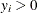is observed). There are also a number of women who did not work at all (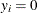is observed). This gives us the following model: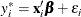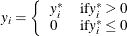where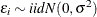. The set of explanatory variables is denoted by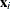.

title1 'Estimating a Tobit model';

data subset;
input Hours Yrs_Ed Yrs_Exp @@;
if Hours eq 0 then Lower=.;
else               Lower=Hours;
datalines;
0 8 9 0 8 12 0 9 10 0 10 15 0 11 4 0 11 6
1000 12 1 1960 12 29 0 13 3 2100 13 36
3686 14 11 1920 14 38 0 15 14 1728 16 3
1568 16 19 1316 17 7 0 17 15
;

/*-- Tobit Model --*/
proc qlim data=subset;
model hours = yrs_ed yrs_exp;
endogenous hours ~ censored(lb=0);
run;


The output of the QLIM procedure is shown in Output 22.2.1.

Output 22.2.1: Tobit Analysis Results

 Estimating a Tobit model

The QLIM Procedure

Model Fit Summary
Number of Endogenous Variables 1
Endogenous Variable Hours
Number of Observations 17
Log Likelihood -74.93700
Maximum Absolute Gradient 1.18953E-6
Number of Iterations 23
Optimization Method Quasi-Newton
AIC 157.87400
Schwarz Criterion 161.20685

Parameter Estimates
Parameter DF Estimate Standard
Error
t Value Approx
Pr > |t|
Intercept 1 -5598.295129 27.692220 -202.16 <.0001
Yrs_Ed 1 373.123254 53.988877 6.91 <.0001
Yrs_Exp 1 63.336247 36.551299 1.73 0.0831
_Sigma 1 1582.859635 390.076480 4.06 <.0001

In the “Parameter Estimates” table there are four rows. The first three of these rows correspond to the vector estimate of the regression coefficients. The last one is called _Sigma, which corresponds to the estimate of the error variance.